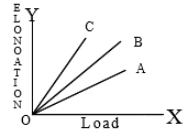A gas undergoes a process in which its pressure P and volume V are related as ${\mathrm{VP}}^{\mathrm{n}}=\mathrm{constant}$. The bulk modulus for the gas in the process is [This question includes concepts from Kinetic Theory chapter]

1. $\frac{P}{n}$

2. ${P}^{1/n}$

3. nP

4. ${P}^{n}$

Subtopic:  Stress - Strain |
To view explanation, please enrol in the course below.
NEET 2021 - Achiever Batch - Aryan Raj Singh

Difficulty Level:

The Young's modulus of a wire of length 'L' and radius 'r' is 'Y'. If length is reduced to L/2 and radius r/2, then Young's modulus will be

1. Y/2

2. Y

3. 2Y

4. 4Y

Subtopic:  Elasticity |
To view explanation, please enrol in the course below.
NEET 2021 - Achiever Batch - Aryan Raj Singh

Difficulty Level:

Three wires A,B,C made of the same material and radius have different lengths. The graphs in the figure show the elongation-load variation. The longest wire is1. A

2. B

3. C

4. All

Subtopic:  Stress - Strain Curve |
To view explanation, please enrol in the course below.
NEET 2021 - Achiever Batch - Aryan Raj Singh

Difficulty Level:

The breaking stress of a wire depends upon

1. material of the wire

2. length of the wire

3. radius of the wire

4. shape of the cross section

Subtopic:  Elasticity |
To view explanation, please enrol in the course below.
NEET 2021 - Achiever Batch - Aryan Raj Singh

Difficulty Level:

The bulk modulus of a spherical object is B. If it is subjected to uniform pressure p, the fractional decrease in radius is

(1)$\frac{P}{B}$

(2) $\frac{B}{3p}$

(3) $\frac{3p}{B}$

(4)$\frac{p}{3B}$

Subtopic:  Stress - Strain |
To view explanation, please enrol in the course below.
NEET 2021 - Achiever Batch - Aryan Raj Singh

Difficulty Level:

The Young's modulus of steel is twice that of brass. Two wires of same length and of same area of cross-section, one of steel and another of brass are suspended from the same roof. If we want the lower ends of the wires to be at the same level, then the weight added to the steel and brass wires must be in the ratio of

(1)1:2

(2)2:1

(3)4:1

(4)1:1

Subtopic:  Stress - Strain |
To view explanation, please enrol in the course below.
NEET 2021 - Achiever Batch - Aryan Raj Singh

Difficulty Level:

Copper of fixed volume V is drawn into wire of length l. When this wire is subjected to a constant force F, the extension produced in the wire is  Δl. Which of the following graphs is a straight line?

(1)Δl versues 1/l

(2)Δl versus l2

(3)Δl versus 1/l2

(4) Δl versus l

Subtopic:  Hooke's Law |
To view explanation, please enrol in the course below.
NEET 2021 - Achiever Batch - Aryan Raj Singh

Difficulty Level:

The following four wires are made of the same material. Which of them will have the largest extension when the same tension is applied?

(1) Length=50cm, diameter=0.5mm

(2) Length=100cm, diameter=1mm

(3) Length=200cm, diameter=2mm

(4) Length=300cm, diameter=3mm

Subtopic:  Hooke's Law |
To view explanation, please enrol in the course below.
NEET 2021 - Achiever Batch - Aryan Raj Singh

Difficulty Level:

When a certain weight is suspended from a long uniform wire, its length increases by one cm. If the same weight is suspended from another wire of the same material and length but having a diameter half of the first one then the increase in length will be -

(1) 0.5 cm

(2) 2 cm

(3) 4 cm

(4) 8 cm

Subtopic:  Hooke's Law |
To view explanation, please enrol in the course below.
NEET 2021 - Achiever Batch - Aryan Raj Singh

Difficulty Level:

The area of cross-section of a wire of length 1.1 metre is 1$m{m}^{2}.$It is loaded with 1 kg. If Young's modulus of copper is $1.1×{10}^{11}N/{m}^{2},$ then the increase in length will be (If $g=10m/{s}^{2}$)

(1) 0.01 mm

(2) 0.075 mm

(3) 0.1 mm

(4) 0.15 mm

Subtopic:  Hooke's Law |
To view explanation, please enrol in the course below.
NEET 2021 - Achiever Batch - Aryan Raj Singh

Difficulty Level: